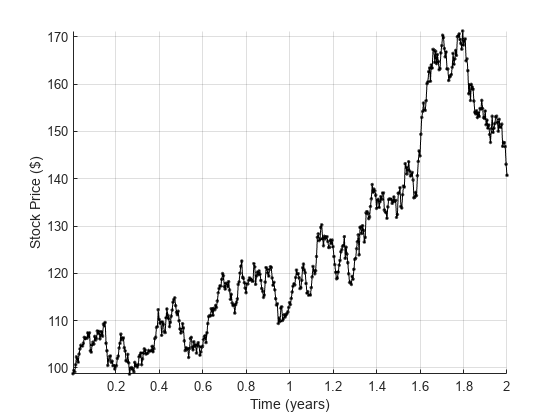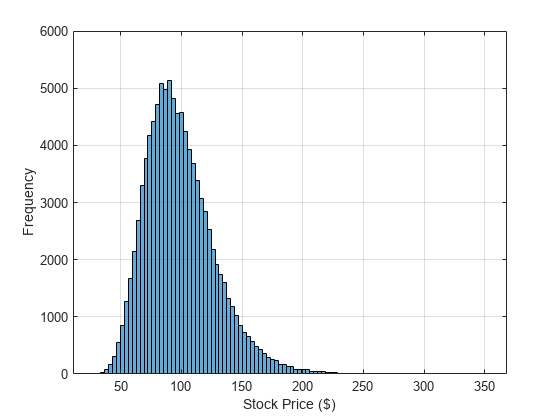# Using GPU ARRAYFUN for Monte-Carlo Simulations

This example shows how prices for financial options can be calculated on a GPU using Monte-Carlo methods. Three simple types of exotic option are used as examples, but more complex options can be priced in a similar way.

This example is a function so that the helpers can be nested inside it.

function paralleldemo_gpu_optionpricing 

This example uses long-running kernels, so cannot run if kernel execution on the GPU can time-out. A time-out is usually only active if the selected GPU is also driving a display.

dev = gpuDevice(); if dev.KernelExecutionTimeout error( 'pctexample:gpuoptionpricing:KernelTimeout', ... ['This example cannot run if kernel execution on the GPU can ', ... 'time-out.'] ); end 

### Stock Price Evolution

We assume that prices evolve according to a log-normal distribution related to the risk-free interest rate, the dividend yield (if any), and the volatility in the market. All of these quantities are assumed fixed over the lifetime of the option. This gives the following stochastic differential equation for the price:whereis the stock price,is the risk-free interest rate,is the stock's annual dividend yield,is the volatility of the price andrepresents a Gaussian white-noise process. Assuming thatis log-normally distributed, this can be discretized to:As an example let's use $100 of stock that yields a 1% dividend each year. The central government interest rate is assumed to be 0.5%. We examine a two-year time window sampled roughly daily. The market volatility is assumed to be 20% per annum. stockPrice = 100; % Stock price starts at$100. dividend = 0.01; % 1% annual dividend yield. riskFreeRate = 0.005; % 0.5 percent. timeToExpiry = 2; % Lifetime of the option in years. sampleRate = 1/250; % Assume 250 working days per year. volatility = 0.20; % 20% volatility. 

We reset the random number generators to ensure repeatable results.

seed = 1234; rng( seed ); % Reset the CPU random number generator. gpurng( seed ); % Reset the GPU random number generator. 

We can now loop over time to simulate the path of the stock price:

price = stockPrice; time = 0; hold on; while time < timeToExpiry time = time + sampleRate; drift = (riskFreeRate - dividend - volatility*volatility/2)*sampleRate; perturbation = volatility*sqrt( sampleRate )*randn(); price = price*exp(drift + perturbation); plot( time, price, '.' ); end axis tight; grid on; xlabel( 'Time (years)' ); ylabel( 'Stock price ($)' );### Running on the GPU To run stock price simulations on the GPU we first need to put the simulation loop inside a helper function:  function finalStockPrice = simulateStockPrice(S,r,d,v,T,dT) t = 0; while t < T t = t + dT; dr = (r - d - v*v/2)*dT; pert = v*sqrt( dT )*randn(); S = S*exp(dr + pert); end finalStockPrice = S; end  We can then call it thousands of times using arrayfun. To ensure the calculations happen on the GPU we make the input prices a GPU vector with one element per simulation. To accurately measure the calculation time on the GPU we use the gputimeit function. % Create the input data. N = 1000000; startStockPrices = stockPrice*ones(N,1,'gpuArray'); % Run the simulations. finalStockPrices = arrayfun( @simulateStockPrice, ... startStockPrices, riskFreeRate, dividend, volatility, ... timeToExpiry, sampleRate ); meanFinalPrice = mean(finalStockPrices); % Measure the execution time of the function on the GPU using gputimeit. % This requires us to store the |arrayfun| call in a function handle. functionToTime = @() arrayfun(@simulateStockPrice, ... startStockPrices, riskFreeRate, dividend, volatility, ... timeToExpiry, sampleRate ); timeTaken = gputimeit(functionToTime); fprintf( 'Calculated average price of$%1.4f in %1.3f secs.\n', ... meanFinalPrice, timeTaken ); clf; hist( finalStockPrices, 100 ); xlabel( 'Stock price ($)' ) ylabel( 'Frequency' ) grid on;  Calculated average price of$98.9563 in 0.283 secs.### Pricing an Asian Option

As an example, let's use a European Asian Option based on the arithmetic mean of the price of the stock during the lifetime of the option. We can calculate the mean price by accumulating the price during the simulation. For a call option, the option is exercised if the average price is above the strike, and the payout is the difference between the two:

 function optionPrice = asianCallOption(S,r,d,v,x,T,dT) t = 0; cumulativePrice = 0; while t < T t = t + dT; dr = (r - d - v*v/2)*dT; pert = v*sqrt( dT )*randn(); S = S*exp(dr + pert); cumulativePrice = cumulativePrice + S; end numSteps = (T/dT); meanPrice = cumulativePrice / numSteps; % Express the final price in today's money. optionPrice = exp(-r*T) * max(0, meanPrice - x); end 

Again we use the GPU to run thousands of simulation paths using arrayfun. Each simulation path gives an independent estimate of the option price, and we therefore take the mean as our result.

strike = 95; % Strike price for the option ($). optionPrices = arrayfun( @asianCallOption, ... startStockPrices, riskFreeRate, dividend, volatility, strike, ... timeToExpiry, sampleRate ); meanOptionPrice = mean(optionPrices); % Measure the execution time on the GPU and show the results. functionToTime = @() arrayfun( @asianCallOption, ... startStockPrices, riskFreeRate, dividend, volatility, strike, ... timeToExpiry, sampleRate ); timeTaken = gputimeit(functionToTime); fprintf( 'Calculated average price of$%1.4f in %1.3f secs.\n', ... meanOptionPrice, timeTaken ); 
Calculated average price of $19.2711 in 0.286 secs.  ### Pricing a Barrier Option This final example uses an "up and out" barrier option which becomes invalid if the stock price ever reaches the barrier level. If the stock price stays below the barrier level then the final stock price is used in a normal European call option calculation.  function optionPrice = upAndOutCallOption(S,r,d,v,x,b,T,dT) t = 0; while (t < T) && (S < b) t = t + dT; dr = (r - d - v*v/2)*dT; pert = v*sqrt( dT )*randn(); S = S*exp(dr + pert); end if S<b % Within barrier, so price as for a European option. optionPrice = exp(-r*T) * max(0, S - x); else % Hit the barrier, so the option is withdrawn. optionPrice = 0; end end  Note that we must now supply both a strike price for the option and the barrier price at which it becomes invalid: strike = 95; % Strike price for the option ($). barrier = 150; % Barrier price for the option ($). optionPrices = arrayfun( @upAndOutCallOption, ... startStockPrices, riskFreeRate, dividend, volatility, ... strike, barrier, ... timeToExpiry, sampleRate ); meanOptionPrice = mean(optionPrices); % Measure the execution time on the GPU and show the results. functionToTime = @() arrayfun( @upAndOutCallOption, ... startStockPrices, riskFreeRate, dividend, volatility, ... strike, barrier, ... timeToExpiry, sampleRate ); timeTaken = gputimeit(functionToTime); fprintf( 'Calculated average price of$%1.4f in %1.3f secs.\n', ... meanOptionPrice, timeTaken ); 
Calculated average price of \$6.8166 in 0.289 secs. 
end 

## SupportGet trial now